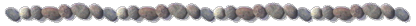Research Interest of Dr. Liao in Nonlinear Waves

(1)  Interaction of Nonlinear Water Wave and Non-uniform Current

In Chapter 15, we illustrate the validity of the homotopy analysis method (HAM) for a complicated nonlinear PDE describing the nonlinear interaction of a periodic traveling wave on a non-uniform current with exponential distribution of vorticity.  In the frame of the HAM, the original highly nonlinear PDE with variable coefficient is transferred into an infinite number of much simpler linear PDEs, which are rather easy to solve.  Physically, it is found that Stokes’ criterion of wave breaking is still correct for traveling waves on non-uniform currents.  It verifies that the HAM can be used to solve some complicated nonlinear PDEs so as to deepen and enrich our physical understanding about some interesting nonlinear phenomena.

For details, please refer to Chapter 15 of Liao’s book: Homotopy Analysis Method in Nonlinear Differential Equations, Higher Education Press & Springer (2012), and the following article:

Cheng, J., Cang, J. and Liao, S.J.: On the interaction of deep water waves and exponential shear currents. Z. angew. Math. Phys.  60: 450 – 478 (2009) [ PDF ]

(2)  Resonance Criterion of Arbitrary Number of Nonlinear Water Waves

In Chapter 16, we verify the validity of the homotopy analysis method (HAM) for a rather complicated nonlinear PDE describing the nonlinear interaction of arbitrary number of traveling water waves.  In the frame of the HAM, the wave resonance criterion for arbitrary number of waves is gained, for the first time, which logically contains the famous Phillips’ criterion for four small amplitude waves.  Besides, it is found for the first time that, when the wave-resonance criterion is satisfied and the wave system is fully developed, there exist multiple steady-state resonant waves, whose amplitude might be much smaller than primary waves so that a resonant wave may contain much small percentage of the total wave energy.  This example illustrates that the HAM can be used as a tool to deepen and enrich our understandings about some rather complicated nonlinear phenomena.

For details, please refer to Chapter 16 of Liao’s book: Homotopy Analysis Method in Nonlinear Differential Equations, Higher Education Press & Springer (2012), and the following article:

Liao, S.J.: On the homotopy multiple-variable method and its applications in the interactions of nonlinear gravity waves. Commun. Nonlinear Sci Numer Simulat, 16:1274 – 1303 (2011) ( arXiv:1005.5539 )